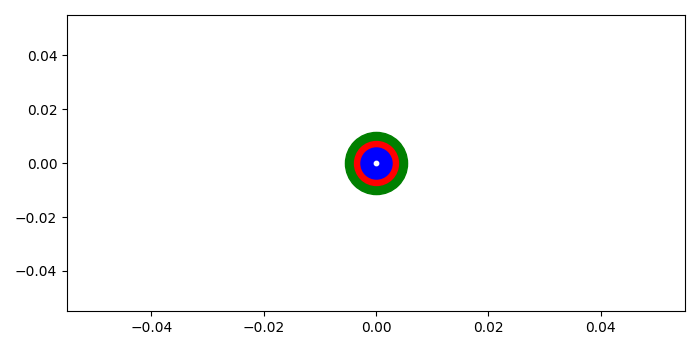# How to make a multicolored point in Matplotlib?

To make a multicolored point in matplotlib, we can take the following steps−

• Initialize two varuables, x and y.
• Use scatter method with x and y data points with green color having marker size 2000.
• Use scatter method with x and y data points with red color having marker size 1000.
• Use scatter method with x and y data points with blue color having marker size 500.
• Use scatter method with x and y data points with white color having marker size 10.
• To display the figure, use show() method.

## Example

from matplotlib import pyplot as plt
plt.rcParams["figure.figsize"] = [7.00, 3.50]
plt.rcParams["figure.autolayout"] = True
x, y = 0, 0
plt.scatter(x, y, c='green', s=2000)
plt.scatter(x, y, c='red', s=1000)
plt.scatter(x, y, c='blue', s=500)
plt.scatter(x, y, c='white', s=10)
plt.show()

## Output